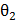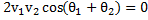# Consider elastic collision of a particle of mass m moving with a velocity u with another particle of the same mass at rest. After the collision the projectile and the stuck particle move in directions making anglesandrespectively with the initial direction of motion. The sum of the anglesa)b)c)d)## Question ID - 50876 :- Consider elastic collision of a particle of mass m moving with a velocity u with another particle of the same mass at rest. After the collision the projectile and the stuck particle move in directions making anglesandrespectively with the initial direction of motion. The sum of the anglesa)b)c)d)3537

(b)

Let particle with mass, move with velocity u,andandbe velocity after collision. Since , elastic collision is one in which the momentum is conserved , we have…..(i)

In perpendicular direction…..(ii)

Also elastic collision occurs only if there is no conversion of kinetic energy into other from, Hence….(iii)

Squaring Esq.(i)and (ii)and adding we getUsing Eq.(iii),we getHenceOrWhen two identical particles collide elastically and obliquely,

One being at rest, then they fly off in mutually perpendicular directions.

Next Question :
 If theof a particle is doubled, then its momentum will a) Remain unchanged b) Be doubled c) Be quadrupled d) Increasetimes